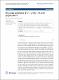## On series solutions of $y'' - f(x)y=0$ and applications2013-03-04
##### Authors
Shivakumar, Pappur N
Zhang, Yang
##### Abstract
AbstractIn this paper, we develop explicit expressions for the Taylor series coefficients in the formal Taylor series solution of the second-order linear differential equation y &#8243; &#8722; f ( x ) y = 0 for a given arbitrary function f ( x ) in terms of initial conditions. As applications, we apply our results to f ( x ) = e x , cos ( x ) and Airy&#8217;s equation and give explicit formulas for the Taylor coefficients of exp ( &#8721; k = 0 &#8734; a k x k ) , which is a long-standing question.
##### Citation
Advances in Difference Equations. 2013 Mar 04;2013(1):47